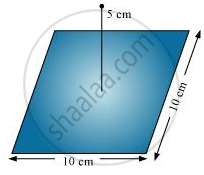# A point charge +10 μC is a distance 5 cm directly above the centre of a square of side 10 cm, as shown in the Figure. What is the magnitude of the electric flux through the square? - Physics

Numerical

A point charge +10 μC is a distance 5 cm directly above the centre of a square of side 10 cm, as shown in the Figure. What is the magnitude of the electric flux through the square? (Hint: Think of the square as one face of a cube with edge 10 cm.)#### Solution

The square can be considered as one face of a cube of edge 10 cm with a centre where charge q is placed. According to Gauss’s theorem for a cube, total electric flux is through all its six faces.

phi_"total" = "q"/in_0

Hence, electric flux through one face of the cube i.e., through the square, phi = phi_"total"/6

= 1/6 q/in_0

Where,

0 = Permittivity of free space

= 8.854 × 10−12 N−1 Cm−2

q = 10 μC = 10 × 10−6 C

∴ phi = 1/6 xx (10 xx 10^-6)/(8.854 xx 10^-12)

= 1.88 × 105 N m2 C−1

Therefore, electric flux through the square is 1.88 × 105 N m2 C−1.

Concept: Electric Flux
Is there an error in this question or solution?

#### APPEARS IN

NCERT Physics Part 1 and 2 Class 12
Chapter 1 Electric Charges and Fields
Exercise | Q 1.18 | Page 47
NCERT Class 12 Physics Textbook
Chapter 1 Electric Charge and Fields
Exercise | Q 18 | Page 47

Share